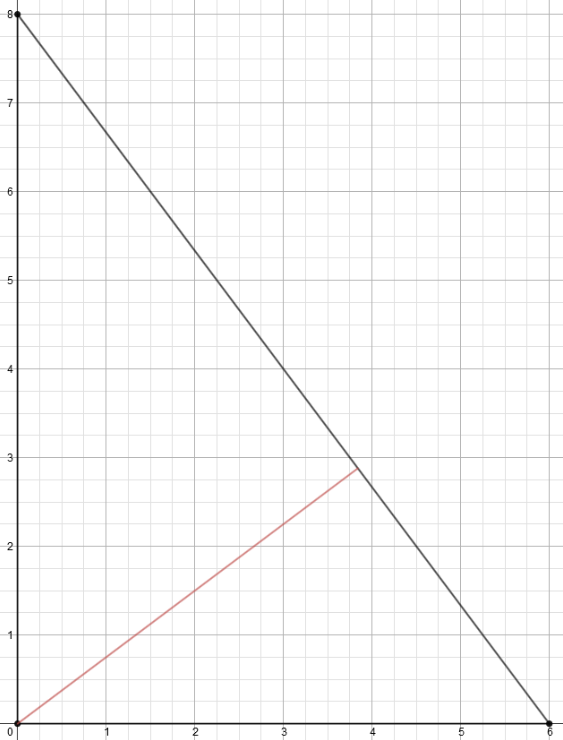Home > High School: Geometry > Expressing Geometric Properties with Equations > Finding the Length of a Right Triangle’s Altitude

# Finding the Length of a Right Triangle’s Altitude

Directions: The black triangle is a right triangle with legs 8 and 6. The vertices are at the points (0,0), (0,8), and (6,0). The red line segment is perpendicular to hypotenuse. Find the length of the red line segment.### Hint

1) What’s the area of the triangle?
2) Would knowing an angle measurement help?
3) Are there similar triangles?
4) Could you find the equation of any of the lines?

There are many methods to finding the answer. Here they are given based on the hint question:

METHOD 1:
The area of a triangle is 0.5(b)(h). In a right triangle, we can use the legs to calculate this, so 0.5(8)(6) = 24. But the red line segment is also the height of the triangle, since it is perpendicular to the hypotenuse, which can also act as a base. So it is also possible to calculate the area by doing 0.5(hypotenuse)(red line).

Pythagoras tells us that the hypotenuse is 10 (6^2 + 8^2 = 10^2), and we already know the area of the triangle is 24, so 24 = 0.5(10)(red line) –> 24 = 5x –> x = 4.8. The red line segment is 4.8.

METHOD 2:
We know that the legs of the right triangle are 6 and 8, so we can use inverse tan to find the base angle. Base angle = arctan(8/6). Base angle = 53.13…

We see that this angle is also in a smaller right triangle formed by the red line segment. In this triangle 6 is the hypotenuse and the red line is the opposite side from the angle we found. So we can do sin(53.13…) = red line/6. Red line = 4.8

*note, you could also do this finding the top angle and using the upper triangle formed by the red line

METHOD THREE:
The red line divides the big triangle into two smaller triangles, both of which are similar to the big triangle. I will work with the bottom triangle and the big triangle. We know they are similar because they both have an angle of 90 degrees and they share the angle at the point (6,0).

The big triangle has:

short leg = 6

long leg = 8

hypotenuse = 10 (Pythagoras)

The small triangle has:

short leg = ?

long leg = red line (x)

hypotenuse = 6

So we can set up a proportion with the long legs and the hypotenuses. x/8 = 6/10 -> 10x = 48, so the red line = 4.8

METHOD FOUR:

The hypotenuse line goes through the points (6,0) and (0,8). Therefore the gradient (slope) is -8/6 = -4/3. The y-intercept is 8. Therefore the equation of the line of the hypotenuse is:

y = -4/3x + 8

Since the red line is perpendicular to the hypotenuse line, its gradient must be 3/4 (negative reciprocal or m*m = -1). Since its y-intercept is 0, the equation of the red line is:

y = 3/4x

To find the point of intersection, we can set the two equations equal to each other:

-4/3x + 8 = 3/4x   (multiply both sides by 12 to eliminate fractions)

-16x + 96 = 9x

96 = 25x

x = 3.84

plug in to find y:

y = 3/4 (3.84)

y = 2.88

So the red line goes from (0,0) to (3.84, 2.88). Now we can use the distance formula to calculate the length of the line segment:

distance^2 = 3.84^2 + 2.88^2

distance^2 = 23.04

distance = 4.8

so the red line = 4.8

Source: Kate Nerdypoo

## Pythagorean Inequality

Directions: Use the whole numbers 1 through 6, at most one time each, to find …